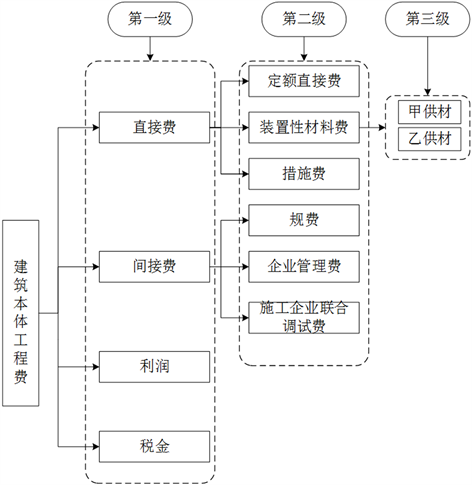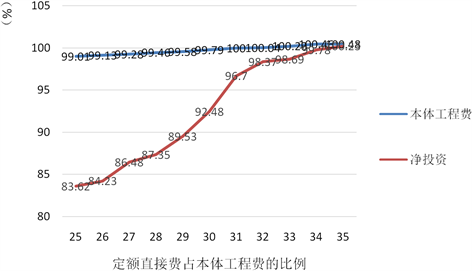# 基于AHP的营改增对变电安装工程造价影响研究Research on the Impact Caused by Replacing the Business Tax with VAT on the Cost of Substation Installation Project on AHP

• 全文下载: PDF(522KB)    PP.44-52   DOI: 10.12677/MM.2018.81005
• 下载量: 552  浏览量: 1,889

Replacing business tax with VAT is a current major tax reform in China. In new circumstances, the original power grid construction project cost and the management of power grid enterprises cannot play its biggest role. Thus to comply with the policy of replacing business tax with VAT and improving the level of the power grid construction project cost management level has been the focus of attention of the power grid enterprises. This paper is based on the power grid project cost management and is guided by the change of the tax calculation. According to the results of AHP method model and the case analysis of power grid enterprises, we put forward some suggestions to implement the project cost management. It helps enterprises grasp the future development trend of construction enterprises in the context of the new macro and adapt to the objective requirements of enterprise management in the transition as soon as possible.

1. 引言

2. 变电安装工程造价理论基础

2.1. 工程造价费用构成

2.2. 工程计价方式调整

1) 税金占比

2) 利润占比

3) 间接费占比

4) 措施费占比

5) 定额直接费

6) 装置性材料

3. 层次分析法的基本原理

3.1. 层次分析法基本原理

3.2. 层次分析法基本步骤

1) 建立递阶层次结构

2) 构造判断矩阵

${a}_{ij}$ 表示专家对指标 ${a}_{i}$${a}_{j}$ 的相对重要性的打分。

3) 计算权重向量

4) 一致性检验

① 根据公式 ${\lambda }_{\mathrm{max}}=\frac{1}{m}{\sum }_{i=1}^{m}\frac{{\sum }_{j=1}^{m}{a}_{ij}{w}_{j}}{{w}_{i}}$ ，计算出判断矩阵的最大特征根；② 利用最大特征根计算判断矩阵的一致性指标 $\text{CI}={\lambda }_{\mathrm{max}}-\frac{m}{m-1}$ ；③ 计算判断矩阵的平均随机一致性指标 $\text{RI}={{\lambda }^{\prime }}_{\mathrm{max}}-\frac{m}{m-1}$ ；④ 随机一致性比例CR为 $\text{CR}=\frac{\text{CI}}{\text{RI}}$ ，当 $\text{CR}<0.10$ 时，即认为判断矩阵具有满意的一致性否则需要调整判断矩阵，使之具有满意的一致性。

4. 基于AHP的变电安装工程造价模型构建

4.1. 评估模型基本假设

4.2. 造价影响模型构建

1) 营改增前各项费用权重确定

① 构造层次结构。架空线路本体工程费的层次结构如图1

② 构造指标的两两比较判断矩阵。

$A=\left[\begin{array}{ccccc}& {u}_{1}& {u}_{2}& {u}_{3}& {u}_{4}\\ {u}_{1}& {a}_{11}& {a}_{12}& {a}_{13}& {a}_{14}\\ {u}_{2}& {a}_{21}& {a}_{22}& {a}_{23}& {a}_{24}\\ {u}_{3}& {a}_{31}& {a}_{32}& {a}_{33}& {a}_{34}\\ {u}_{4}& {a}_{41}& {a}_{42}& {a}_{43}& {a}_{44}\end{array}\right]$

${a}_{ij}$ 表示专家对指标 ${a}_{i}$${a}_{j}$ 的相对重要性的打分。将所得到的判断矩阵A进行量化，利用比例标度法经过专家打分，比较各指标之间的重要程度，写出判断矩阵A，见表1

③ 利用几何平均值法排序权重。

④ 一致性检验。Figure 1. The architecture of the cost of the EngineeringTable 1. The judgment matrix A of the first level index

2) 营改增后各项费用调整系数

① 营改增后，定额直接费中人工费不除税，并未发生变化，消耗性材料费和机械费按不同税率除税，综合编制基准期价差影响测算，营改增后定额水平下降5.8%左右，即调整系数为0.942。装置性材料费中甲供材不作调整，即甲供材调整系数为1；乙供材按13%除税价计入建筑安装工程费，调整系数为0.885。

② 措施费的费用调整系数应由取费费率的变化与取费基数的相乘可得。变电安装工程措施费一般包括冬雨季施工增加费、夜间施工增加费、施工工具用具使用费、临时设施费、施工机构迁移费、安全文

③ 间接费包括规费、企业管理费和施工企业配合调试费。规费以人工费为基数，营改增后规费取费基数和取费费率均未发生变化，其调整系数为1。企业管理费以人工费为基数；施工企业配合调试费以直接费为基数，最终分析得到间接费的调整系数为0.99。

④ 利润按《电力建设工程定额估价表》要求计取，利润 = (直接费 + 间接费) × 6.67%，按本研究中数学模型基础费用结构分析，营改增后利润 = (定额直接费 + 甲供材 + 乙供材 + 措施费 + 规费 + 企管 + 施配费) × 6.67%。

⑤ 税金按《电力建设工程定额估价表》要求计取，税金 = (定额直接费 + 乙供材 + 措施费 + 规费 + 企管 + 施配费 + 利润) × 11%，即甲供材不作为安装工程费的取费基数。

3) 营改增后各项费用权重确定Table 2. The weight list of the expenses before the increase of the campTable 3. The analysis model of the influence of the cost of substation installation engineering

4.3. 造价影响敏感性分析

5. 实例分析Figure 2. The corresponding cost diagram of different quota direct charge ratioTable 4. The statistical table of the influence of the change of camp on the cost of the substation installation project

6. 结论

1) 积极转变管理思维，引领企业发展方向

2) 合理开展税务筹划，规避税务管控风险

3) 着力强化会计核算，更新财务管理体制

4) 持续优化业务结构，提升企业管理水平

  刘琳雁. “营改增”的必要性和中小企业的现状浅析[J]. 中国集体经济, 2015(25): 49-50.  李金宇, 陈明. 增值税与营业税合并的现实分析[J]. 商, 2015(29): 181-182.  孙伟, 王剑. “营改增”实施的必要性及对我国第三产业经济的影响[J]. 科技与管理, 2015, 17(1): 110-113.  张新, 安体富. 营改增减收效应分析[J]. 税务研究, 2013(10): 9-13.  田志伟, 胡怡建. 营改增对财政经济的动态影响一基于CGE模型的分析[J]. 财经研究, 2014, 40(2): 4-18.  詹敏. 建筑业“营改增”建安费用扣减问题管见[J]. 财会月刊, 2015(19): 49-51.  滕小芳, 张春雷. “营改增”导致部分企业税负增加的原因及应对[J]. 北方经贸, 2014(4): 77-79.